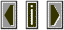Eigentonean acoustical resonance or standing wave in an enclosed space caused by parallel surfaces.  the distance between the surfaces (usually walls) is half the wavelength.  for example if the parallel walls are 11 feet apart the fundamental frequency of the resonance would be (roughly) 51 Hz. (calculation:  (2 X distance) / speed of sound = time for one cycle. frequency = 1 / period (cycle duration).  all this assumes that the surfaces are sound-reflective.  Eigentones are one of the prime reasons for using angles other than 90º or 270º in concert halls.